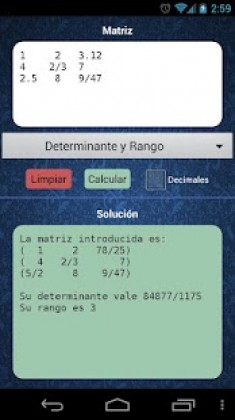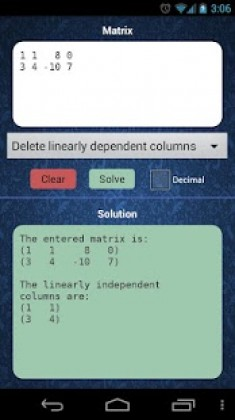# Matroid 1.4 Apk Paid latest

event_note05 Jan 2018
android Apps, Education

Matroid 1.4 Apk Paid latest is a Education Android app

Matroid is a Education android app made by Fernando Enzo Guarini that you can install on your android devices an enjoy !
Matroid is the first application of matrices for Android that lets you:

» Add, subtract and multiply matrices.
» Calculate determinants, ranges and inverse matrices.
» Delete rows and columns linearly dependent of a matrix.
» Solve systems of linear equations determined and undetermined, by obtaining their solutions based on parameters if necessary.

And all of this, always working with both fractions and decimal numbers of completely arbitrary precision to obtain results as accurate as desired.
Furthermore, Matroid has no limits on the size of matrices!

This application is constantly evolving, and it is anticipated in future updates calculating characteristic polynomials and eigenvalues.

In this free version of the application, options for multiplying matrices, get the inverse and solve systems of equations are not available.Matroid ApkMatroid Apk

Whats New:
» Add, subtract and multiply matrices.
» Calculate determinants, ranges and inverse matrices.
» Delete rows and columns linearly dependent of a matrix.
» Solve systems of linear equations determined and undetermined, by obtaining their solutions based on parameters if necessary.